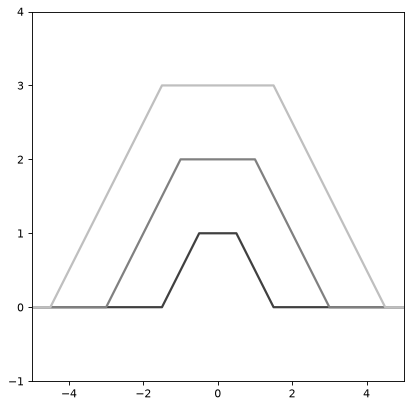Trapezoid1D¶

class astropy.modeling.functional_models.Trapezoid1D(amplitude=1, x_0=0, width=1, slope=1, **kwargs)[source]

One dimensional Trapezoid model.

Parameters: Other Parameters: amplitude : float Amplitude of the trapezoid x_0 : float Center position of the trapezoid width : float Width of the constant part of the trapezoid. slope : float Slope of the tails of the trapezoid fixed : a dict, optional A dictionary {parameter_name: boolean} of parameters to not be varied during fitting. True means the parameter is held fixed. Alternatively the fixed property of a parameter may be used. tied : dict, optional A dictionary {parameter_name: callable} of parameters which are linked to some other parameter. The dictionary values are callables providing the linking relationship. Alternatively the tied property of a parameter may be used. bounds : dict, optional A dictionary {parameter_name: value} of lower and upper bounds of parameters. Keys are parameter names. Values are a list or a tuple of length 2 giving the desired range for the parameter. Alternatively, the min and max properties of a parameter may be used. eqcons : list, optional A list of functions of length n such that eqcons[j](x0,*args) == 0.0 in a successfully optimized problem. ineqcons : list, optional A list of functions of length n such that ieqcons[j](x0,*args) >= 0.0 is a successfully optimized problem.

Examples

import numpy as np
import matplotlib.pyplot as plt

from astropy.modeling.models import Trapezoid1D

plt.figure()
s1 = Trapezoid1D()
r = np.arange(-5, 5, .01)

for factor in range(1, 4):
s1.amplitude = factor
s1.width = factor
plt.plot(r, s1(r), color=str(0.25 * factor), lw=2)

plt.axis([-5, 5, -1, 4])
plt.show()

()Attributes Summary

 amplitude input_units This property is used to indicate what units or sets of units the evaluate method expects, and returns a dictionary mapping inputs to units (or None if any units are accepted). param_names slope width x_0

Methods Summary

 evaluate(x, amplitude, x_0, width, slope) One dimensional Trapezoid model function

Attributes Documentation

amplitude = Parameter('amplitude', value=1.0)
input_units

This property is used to indicate what units or sets of units the evaluate method expects, and returns a dictionary mapping inputs to units (or None if any units are accepted).

Model sub-classes can also use function annotations in evaluate to indicate valid input units, in which case this property should not be overridden since it will return the input units based on the annotations.

param_names = ('amplitude', 'x_0', 'width', 'slope')
slope = Parameter('slope', value=1.0)
width = Parameter('width', value=1.0)
x_0 = Parameter('x_0', value=0.0)

Methods Documentation

static evaluate(x, amplitude, x_0, width, slope)[source]

One dimensional Trapezoid model function